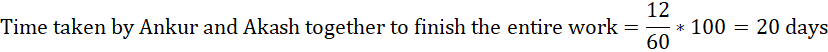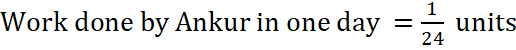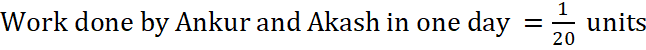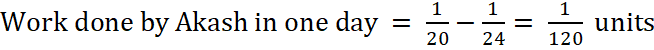Question of The Day04-07-2022

Ankur started a work and finished 75% of it in 18 days. If Ankur and Akash started to work together, they could complete 60% of the work in 12 days. Find the time taken by Akash to complete the work.

Correct Answer : e ) 120 days

Explanation :

According to question

Ankur can finish 75% of the work in 18 daysAnkur and Akash together can finish 60% of work in 12 daysNow from here, there are two approaches:

Basic Method:Thus, time taken by Akash to complete the work = 120 days

Shortcut:

Let us suppose that total units of work is 120.

Ankur completes 120/24 = 6 units of work in a day.

Akash and Ankur together complete 120/20 = 6 units of work in a day.

If Akash worked alone, he will do 6 – 5 = 1 unit of work.

Time taken by Akash to complete 120 units of work is 120 days.

Hence, (e) is the correct answer.1